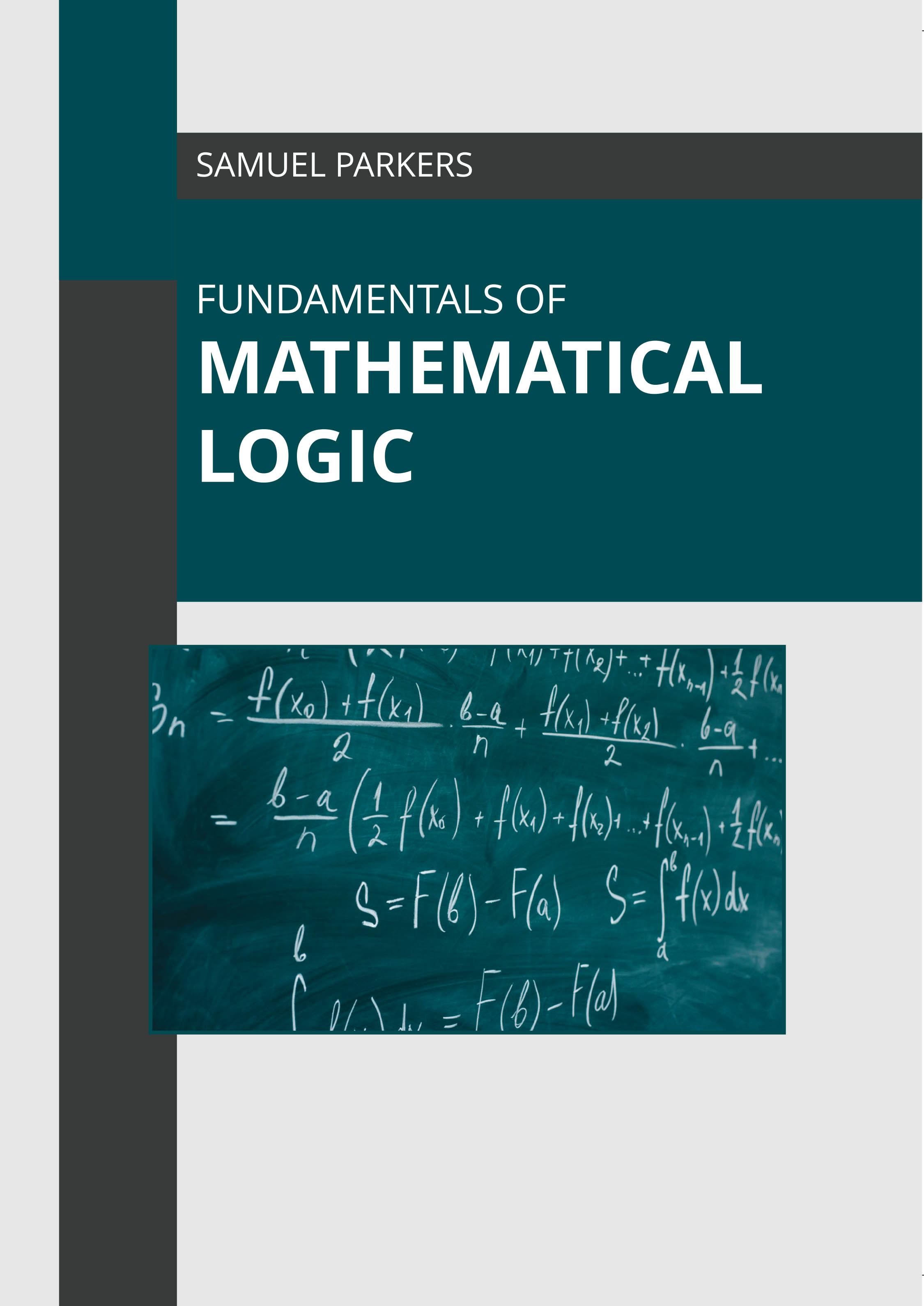# Books /Fundamentals of Mathematical Logic

BROWSE BY

### Fundamentals of Mathematical Logic9781639892280

Author

Samuel Parkers

English

##### Publication Year

(2022)

Category :

Mathematics and Statistics - Numerical Analysis

\$ 155.95

##### Description

The sub-field of mathematics that focuses on identifying the applications of formal logic to mathematics is known as mathematical logic. It is also known as symbolic logic or formal logic. It is concerned with the study of expressive and deductive power of formal systems. Some of the formal logical systems are first-order logic, nonclassical and modal logic, algebraic logic and other classical logics. The discipline is divided into four areas. These are model theory, proof theory, set theory and recursion theory. The field is closely related to theoretical computer science and foundations of mathematics. The field finds its applications in other disciplines such as physics, biology, economics, metaphysics, law and morals, and psychology. This book explores all the important aspects of related to this discipline in the present day scenario. Different approaches, evaluations, methodologies and studies on mathematical logic have been included herein. As this field is emerging at a rapid pace, the contents of this book will help the readers understand the modern concepts and applications of the subject.